Next: 14. Macetes Up: 2. User's Guide Previous: 12. The Proof System

Subsections

# 13.1 Motivation

One of the goals of the IMPS system is to assist users in producing formal proofs in which the number of steps and the kinds of justification used for each step are close to those of a rigorous and detailed (but informal) proof. One of the main reasons that formal proofs are usually so long is that many proof steps involve replacement of an occurrence of a term t1 with a term t2 having the same value as t1 and which is obtained from t1 by a variety of theory-specific transformations. A formal proof might require hundreds of steps to justify such a replacement. In IMPS, the simplifier is designed to handle many of these inferences directly without user intervention and to bundle them into a single inference step. The simplifier accomplishes this as follows:

• By invoking a variety of theory-specific transformations on expressions, such as rewrite rules and simplification of polynomials (given that the theory has suitable algebraic structure, such as that of a field);

• By simplifying expressions based on their logical structure; and

• By discharging the great majority of definedness and sort-definedness assertions needed to apply many forms of inference.

The simplifier can be invoked directly by the user as an IMPS command. As such it is an exceedingly powerful tool. More significantly, the simplifier is a central element in the IMPS inference architecture, since it is routinely invoked by other commands.

The simplifier can be viewed as a function of a context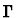and an expression e which returns an expression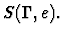The following condition serves as the correctness requirement for the simplifier: For any context(in a theory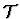) and expression e,andmust together entail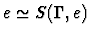. That is to say, either e and e' are both defined and share the same denotation, or else they are both undefined.

# 13.2 Implementation

The simplifier is a highly recursive procedure. For instance, simplification of a compound expression may require prior simplification of all its components (that this is not always true reflects the fact that the simplification procedure for an expression depends on the constructor of the expression). Simplification of individual expression components is done with respect to the local context of that component in the expression. Thus, for instance, in simplifying an implication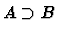, A may be assumed true in the local context relative to which B is simplified. Similarly, in simplifying the last conjunct C of a ternary conjunction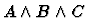, A and B may be assumed in the local context. On the other hand, when a variable-binding operator is traversed, and there are context assumptions in which the bound variable occurs free, then the simplifier must either rename the bound variable or discard the offending assumptions. The strategy of exploiting local contexts is discussed in .

At any stage in this recursive descent, certain theory-specific procedures may be applied to a subexpression of the original expression or to a semantically equivalent form of the original expression. These procedures are called transforms. A transform T takes two arguments, a contextand an expression e, and returns two values, an expression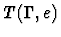and a set of formulas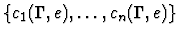called convergence requirements. To apply a transform T to a contextand an expression e means to do one of three things:

• If the simplifier can determine that all the convergence requirements are met in, replace e with.

• If the simplifier can determine that one of the convergence requirements fails in, replace e with an undefined expression of the same sort as e.

• Otherwise, leave e as it is.

This process is sound provided, for every contextand expression e, the following conditions hold:

• If all the convergence requirements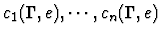are true in, then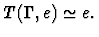• If any of the convergence requirements is false in, thenis undefined.

Each theory has a table of transforms indexed on two keys: (1) a constructor, a quasi-constructor, or the atom nil and (2) a constant or the atom no-defined-constant. The simplifier determines what transforms in the table to apply to an expression as follows:

• It first computes the expression's lead constructor. This is the quasi-constructor of the expression if it has one. Otherwise, it is the constructor of the expression (which is the atom nil for variables and constants).

• It then computes the given expression's lead constant. This is the first constant in a left-to-right traversal of the expression tree, if there is one, or no-defined-constant otherwise. Thus, the lead constant of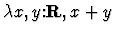is +.

• Finally, it applies, in a nondeterministic order, the transforms in the table whose keys are the same as the expression's lead constructor and lead constant.

# 13.3 Transforms

The transforms used by the IMPS simplifier include:

1.
Algebraic simplification of polynomial expressions.
2.
A decision procedure for linear inequalities, based on the variable elimination method used in many other theorem provers.
3.
Rewrite rules for the current theory, or for certain theories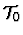for which IMPS can find interpretations frominto).

The framework for applying rewrite rules is entirely general, and uses pattern matching and substitution in a familiar way. By contrast, the transforms that perform algebraic manipulation use specially coded procedures; they are applied to expressions in a way that may not be easily expressed as patterns. Nevertheless, their validity, like the validity of rewrite rules, depends on theorems, many of which are universal, unconditional equalities (e.g., associative and commutative laws).

# 13.4 Algebraic Processors

Instead of providing a fixed set transforms for manipulating expressions in a limited class of algebraic structures, we have implemented a facility for automatically generating and installing such transforms for general classes of algebraic structures. This is possible since algorithmically the transforms are the same in many cases; only the names have to be changed, so to speak. The algebraic manipulation transform is one component of a data structure called an algebraic processor.

An algebraic processor has either two or three associated transforms. There is one transform for handling terms of the form f(a,b) where f is one of the algebraic operations in the processor definition. A separate transform handles equalities of algebraic expressions. Frequently, when the structure has an ordering relation, there is a third transform for handling inequalities of algebraic expressions.

When an algebraic processor is created, the system generates a set of formulas which must hold in order for its manipulations to be valid. The processor's transforms are installed in a theoryonly if the system can ascertain that each of these formulas is a theorem of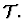The user builds an algebraic processor using a specification such as

   (def-algebraic-processor FIELD-ALGEBRAIC-PROCESSOR
(language fields)
(base ((operations
(+ +_kk)
(* *_kk)
(- -_kk)
(zero o_kk)
(unit i_kk))
commutes)))

This specification has the effect of building a processor with a transform for handling terms of the form f(a,b), where f is one the arithmetic functions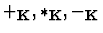. For example, the entry (+ +_kk) tells the transform to treat the function symbol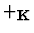as addition. The transforms are installed in the theory fields by the specification
   (def-theory-processors FIELDS
(algebraic-simplifier
(field-algebraic-processor *_kk +_kk -_kk))
(algebraic-term-comparator field-algebraic-processor))

When this form is evaluated, IMPS checks that the conditions for doing algebraic manipulation do indeed hold in the theory fields.

# 13.5 Hints and Cautions

1.
Though simplification is a powerful user command, there are several reasons why you do not want to invoke it indiscriminately at every sequent node:

• If the sequent node context is very large or the expression is large (a fact which may be masked by the presence of quasi-constructors), simplification may take considerable time.

• Simplification may transform an expression into a form which may be easier for IMPS to work with, but may be harder for you to visualize. For instance, the expression x-y simplifies to x+[-1]y.

2.
The simplifier may at times appear to exhibit temperamental'' behavior. At one extreme, it may seem to the user that the simplifier does too little or that it has ignored an assumption that would have immediately grounded the sequent. At the other extreme, the simplifier may do too much work, reducing the assertion in unintuitive or unusable ways.

What may be especially infuriating to the user is that the presence of additional assumptions may actually cause the simplifier to return a much less usable answer. For instance, in the theory h-o-real-arithmetic, the simplifier will reduce the formula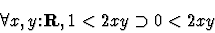to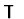. However,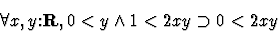simplifies to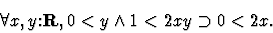This discrepancy results from its factoring the positive y in 0<2 x y before applying the decision procedure for linear inequalities.Next: 14. Macetes Up: 2. User's Guide Previous: 12. The Proof System